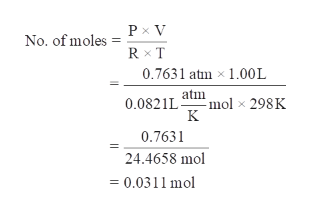# A 4.00 g sample of a mixture of BaO and CaO is placed in a 1.00 L vessel containing CO2 at a pressure of 730 torr and a temperature of 298 K. The CO2 reactions with both oxide reagents to form BaCO3 and CaCO3. When the reaction is complete, the pressure of the remaining CO2 is 150 torr. a. Calculate the total number of moles of CO2 that has reacted. b. Calculate the mass percentage of CaO in the initial mixture.

Question
72 views

A 4.00 g sample of a mixture of BaO and CaO is placed in a 1.00 L vessel containing CO2 at a pressure of 730 torr and a temperature of 298 K. The CO2 reactions with both oxide reagents to form BaCO3 and CaCO3. When the reaction is complete, the pressure of the remaining CO2 is 150 torr. a. Calculate the total number of moles of CO2 that has reacted. b. Calculate the mass percentage of CaO in the initial mixture.

check_circle

Step 1

First we have to calculate the reacted pressure of CO2 gas in the given reaction:-

The initial pressure of CO2 in the reaction is given as 730 torr.

The remaining pressure of CO2 gas after the reaction is given as 150 torr.

So, the reacted pressure of CO2 gas in the given reaction is 580 torr.

Step 2

Now, we will find the no. of moles of reacted CO2 gas reacted in the given reaction: -

Step 3

The volume of container is 1.00 L.

The temperature of the reaction is 298 K.

H...help_outlineImage TranscriptioncloseP x V No. of moles R T 0.7631 atm 1.00L atm mol x 298K 0.0821L 0.7631 24.4658 mol = 0.0311 mol fullscreen

### Want to see the full answer?

See Solution

#### Want to see this answer and more?

Solutions are written by subject experts who are available 24/7. Questions are typically answered within 1 hour.*

See Solution
*Response times may vary by subject and question.
Tagged in

### Solutions